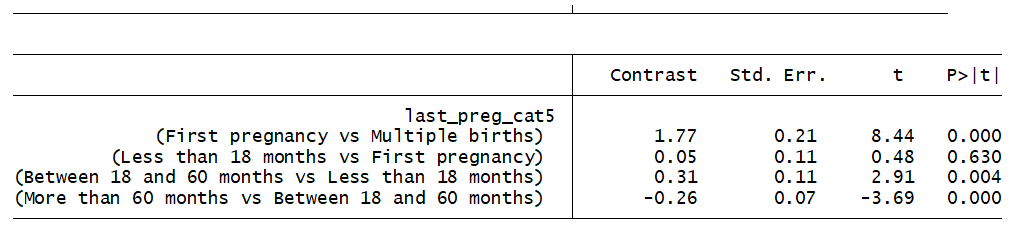Recoding a Variable from a Survey Question to Use in a Statistical Model

by

Survey questions are often structured without regard for ease of use within a statistical model.

Take for example a survey done by the Centers for Disease Control (CDC) regarding child births in the U.S. One of the variables in the data set is “interval since last pregnancy”. Here is a histogram of the results.Reading the survey’s user guide it turns out the variable contains both nominal and continuous data. The continuous data has values from 4 to 300 months.  The nominal data is “no previous pregnancy” which has the value of 888 and “plural delivery” equal to the value of 3.

It’s not possible to use a variable in a statistical analysis if it is a combination of nominal and continuous data. This data could be an important predictor in an explanatory analysis on birth weight. How can we use it?

One strategy is to create a categorical predictor.

Two categories are easy to determine. One category would represent first pregnancy (no previous pregnancy) and the second would represent multiple births (plural delivery).

How should the balance of the data be categorized? One strategy is to aggregate data based on theory.

Research conducted by Dr. Agustin Conde-Agudelo and colleagues found the risk for low birth weight increased by 3.3% for each month less than 18 months. For each month between pregnancies longer than five years the risk of low birth weight increased by 0.6% to 0.9%.

Using the information from this study there is a theoretical reason to split the data into five categories.

1. Multiple births
2. First pregnancy
3. Less than 18 months since last pregnancy
4. Between 18 and 60 months since last pregnancy
5. Greater than 60 months since last pregnancy

Regressing birth weight in pounds on the new categorical variable using a 3,000-observation random subsample of the data produced the following results.Running a pairwise comparison of adjacent categories produces results that concur with the research done by Dr. Conde-Agudelo.The bottom line: when a variable is a combination of continuous and nominal data, use theory to determine the aggregation of the data when creating a new categorical predictor.Four Critical Steps in Building Linear Regression Models
While you’re worrying about which predictors to enter, you might be missing issues that have a big impact your analysis. This training will help you achieve more accurate results and a less-frustrating model building experience.